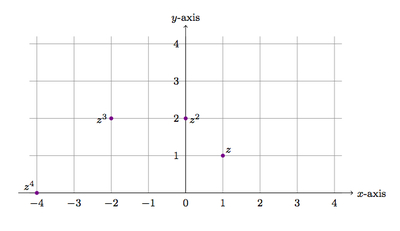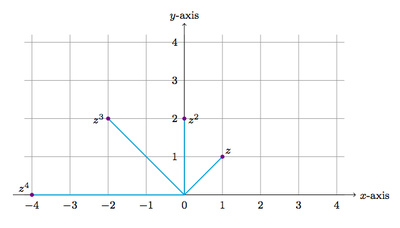# Powers of a complex number

Alignments to Content Standards: N-CN.A.2

1. Let $z = 1 + i$ where $i^2 = -1$. Calculate $z^2, z^3,$ and $z^4$.
2. Graph $z, z^2, z^3,$ and $z^4$ in the complex plane. What do you notice about the positions of these numbers?
3. What is $z^{100}$? Explain.

## IM Commentary

The goal of this task is to practice making calculations with complex numbers and to visualize what happens when two complex numbers are multiplied. The calculations require using the commutative and distributive properties as well as the fact that $i^2 = -1$. In order to calculate $(1+i)^{100}$ students will need to use properties of exponents.

From the geometric perspective, when complex numbers are multiplied their distance from (0,0) is multiplied and the angles that they make with the positive real axis are added. These properties are taken up further in N-CN.4 and N-CN.5 and so the goal here is to introduce students to these ideas in a concrete situation.

## Solutions

Solution: 1

1. For $z^2$ we have

\begin{align} (1+i)^2 &= 1 + 2i + i^2 \\ &= 1 + 2i -1 \\ &= (1-1) + 2i \\ &= 2i. \end{align}

The first step uses the distributive property, the second step uses the fact that $i^2 = -1$, and the third step uses the commutative property of addition.

For $z^3$ we use our calculation above and the fact that $z^3 = z^2 \times z$:

\begin{align} (1+i)^3 &= (1+i)^2 \times (1 + i) \\ &= 2i \times (1 + i) \\ &= 2i + 2i^2\\ &= -2 + 2i. \end{align}

The third line uses the distributive property of multiplication over addition and the last step uses the fact that $i^2 = -1$. Finally, for $z^4$ we have

\begin{align} (1+i)^4 &= (1+i)^3 \times (1 + i) \\ &= (-2+2i) \times (1 + i) \\ &= -2 - 2i +2i +2i^2\\ &= -4. \end{align}

The third equality uses the distributive property and the last step uses the fact that $i^2 = -1$.

2. Writing each complex number $z$ as $x + iy$ we can plot $z, z^2, z^3,$ and $z^4$ in the $x$-$y$ plane as shown below:One thing we notice about these points is that each successive power of $z$ is further from (0,0). The second thing we notice is that the direction from (0,0) to the powers of $z$ changes with the power. In fact, the direction from (0,0) to each successive power of $z$ is 45 degrees further in the counterclockwise direction. To see this more clearly, in the following picture the path from (0,0) to the different powers of $z$ has been highlighted:3. We found in part (a) that $z^4 = -4$. So we have

\begin{align} z^{100} &= (1+ i)^{100} \\ &= \left((1 + i)^4\right)^{25} \\ &= (-4)^{25} \\ &= -4^{25}. \end{align}

Solution: 2 Looking for patterns for part (c)

In order to find $(1+i)^{100}$, we begin by calculating some smaller powers of $(1+i)$ and then look for a pattern. We find

\begin{align} (1+i)^2 &= 2i\\ (1+i)^3 &= -2 + 2i\\ (1+i)^4 &= -4\\ (1+i)^5 &= -4 - 4i\\ (1+i)^6 &= -8i\\ (1+i)^7 &= 8-8i\\ (1+i)^{8} &= 16. \end{align}
One efficient way to make these calculations is to build upon previous results: for example, to find $(1+i)^3$, we use the result for $(1+i)^2$:
\begin{align} (1+i)^3 &= (1+i)(1+i)^2 \\ &= (1+i)(2i)\\ &= 2i^2 + 2i\\ &= -2 + 2i. \end{align}
We notice that the 4th and 8th powers of $1+i$ are real numbers. More generally, any power of $1+i$ which is a multiple of 4 will be a real number. Using the law of exponents, we can find $(1+i)^{100}$ by dividing it into groups of 4
\begin{align} (1+i)^{100} &= \left((1+i)^4\right)^{25} \\ &= (-4)^{25}\\ &= -4^{25}.\\ \end{align}